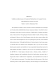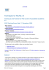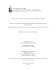# Estimation of period and Q of the Chandler wobble

## Transcription

Estimation of period and Q of the Chandler wobble
```Geophys. J. lnt. (1996) 127, 693-702
Estimation of period and Q of the Chandler wobble
M.Furuya' and B. F. Chao2
'DPpartmnt of Earth and Planetary Physics, University of Tokyo, Tokyo 1 13, Japan
'Jhoratoryfor Terrestrial Physics, NASA Goddard Space Flight Center, Greenbelt, M D 20771, USA
Accepted 1996 July 31. Received 1996 July 25; in original form 1995 December 25
SUMMARY
The period P and Q-value of the Chandler wobble are two fundamental functionals of
the Earth's internal physical properties and global geodynamics. We revisit the problem
of the estimation of P and Q, using 10.8yr of modern polar motion as well as
contemporary atmospheric angular momentum (AAM) data. We make full use of the
knowledge that AAM is a major broad-band excitation source for the polar motion.
We devise two optimization criteria under the assumption that, after removal of
coherent seasonal and long-period signals, the non-AAM excitation is uncorrelated
with the AAM. The procedures lead to optimal estimates for P and Q. Our best
estimates, judging from comprehensive sets of Monte Carlo simulations, are P =
433.7 f 1.8 (lo)days, Q = 4 9 with a lo range of (35, 100).In the process we also obtain
(as a by-product) an estimate of roughly 0.8 for a 'mixing ratio' of the invertedbarometer (IB) effect in the AAM pressure term, indicating that the ocean behaves
nearly as IB in polar motion excitation on temporal scales from months to years
Key words: atmospheric angular momentum, Chandler wobble, period, polar motion,
Q-factor.
1 INTRODUCTION
The free Eulerian motion in the Earth's rotation, known as
the Chandler wobble, was discovered in astrometric data by
S . Chandler over 100 years ago. It has a period P of about 14
months as viewed from the terrestrial reference frame, and a
finite quality factor Q due to inevitable energy dissipation.
Chandler P and Q are two of the fundamental functionals for
global geodynamics. Observations of their values are useful
constraints in the inference of physical properties of the Earth's
interior (e.g. Smith & Dahlen 1981; Okubo 1982; Zschau 1986):
P depends sensitively on the mantle elasticity and anelasticity
structure, the extent to which the fluid core is decoupled from
the mantle, and how close the pole tide is to equilibrium,
whereas Q contains information about the budget and processes of kinetic energy dissipation by the oceans, mantle
anelasticity, and coremantle coupling.
The 'International Latitude Service (ILS) began routine
monitoring of the Earth's polar motion around 1900. Estimates
of Chandler P and Q were made, once reasonably accurate
data had been accumulated. Observationally, the Chandler
wobble is a major component in the polar motion, along with
the annual wobble and a polar drift. It has continued to exist
since its discovery, and has exhibited a complex behaviour. Its
amplitude varied slowly with an apparent 40 year modulation
from a few tens to a few hundreds of milliarcseconds (mas).
The spectrum exhibited multiple-component fine structure and
01996 RAS
a phase reversal around 1930. As its exact nature and excitation
sources remain far from fully understood, this complex behaviour has posed great challenges to attempts to estimate
Chandler P and Q.
Table 1 includes past estimates of P and Q using ILS data,
by Jeffreys (1940, 1968), Ooe (1978), Wilson & Haubrich
(1976), and Wilson & Vicente (1980, 1990). Two approaches
were taken. The approach devised by Jeffreys (and later
followed by Wilson & Haubrich 1976 and Wilson & Vicente
1990) seeks minimum variance for the excitation in a maximum-likelihood scheme. The approach used by Ooe (1978)
and Wilson & Vicente ( 1980) adopts autoregressive-movingaverage modelling for the observed polar motion. Both
approaches assume Gaussian random excitations. This
assumption, however, is hardly realistic. Physically, under the
conservation of angular momentum, the excitation of Chandler
wobble requires geophysical processes that involve mass movement in or on the Earth (e.g. Munk & MacDonald 1960). All
geophysical processes are characterized by certain statistical
properties. There is no a priori reason to expect randomness
or 'Gaussian-ness'; the 'systematic' behaviour in the ILS observations described above attests to that fact.
To identify the Chandler excitation sources has remained an
outstanding geophysical problem for decades. Only recently
has the search been partially successful, as the variation of the
atmospheric angular momentum (AAM) was found to be
responsible for a major portion of the polar motion excitation,
693
694
M . Furuya and B. F. Chao
Table 1. Summary of past and present estimates for the free Chandler wobble period and
uality factor Q
- and l o uncertainty.
Study
Period (days)
. - .
Q (range)
Data (length in yr)
Jeffreys (194)
446.7 i 6.8
46 (37,60)
ILS (42)
Jeffreys (1968)
433.2 f 3.4
61 (37, 193)
ILS (68)
Wilson & Haubrich (1976)
434.0 -t 2.5
100 (50,400)
ILS (70)
Ooe (1978)
434.8 f 2.0
96 (50,300)
ILS (76)
Wilson & Vicente (1980)
433.3 i 3.6
175 (48, 1OOO)
ILS (78)
Wilson & Vicente (1990)
433.0 f 1.1
179 (74,789)
ILS+BM (86)
Kuehne et al. (1996)
439.5 i 1.2
---
Space93+AAM (9)
This Study
433.7 f 1.8
49 (35,
especially on intraseasonal scales (Eubanks et al. 1988; Chao
1993; Kuehne, Wilson & Johnson 1993). Furuya, Hamano &
Naito (1996) have found further evidence that suggests a
strong influence of the wind term of AAM on the polar motion
in and near the Chandler band.
These recent advances in the understanding of polar motion
excitation have motivated us to revisit the estimation problem
for Chandler P and Q . We shall not assume that the excitation
source satisfies the neat statistical condition of being random
and Gaussian. Rather, making full use of the knowledge that
the AAM is a major excitation source for the polar motion,
we devise two different estimators based on simple optimization
criteria. The only assumption we will make is that the nonAAM excitation is uncorrelated with AAM (and we ‘define’
our AAM to conform to this condition, see below). Both
estimators prove to be efficient and asymptotically unbiased;
our adopted results (judged from a series of Monte Carlo
experiments) are given in Table 1. It should be pointed out
that one of our estimators is in principle equivalent to a recent,
independent work by Kuehne et al. (1996; but see below),
whose P estimate is also listed in Table 1.
2 FORMULATION
The polar motion is a 2-D quantity; expressing it in complex
form greatly facilitates the numerical manipulation (Munk &
MacDonald 1960). Thus, as customary, polar motion is
expressed as m = x + i y in radians, where x is the component
of pole position along the Greenwich Meridian and y is that
along 90”E. The polar motion excitation function as well as
the AAM terms will all be similarly expressed in the same
terrestrial coordinates. In the corresponding power spectrum,
positive frequency means prograde motion while negative
2.1 The problem
In order to utilize AAM, we must first ‘define’ our AAM. The
AAM consists of the wind (‘motion’) term and the pressure
(‘mass’) term; both are global integrals with respect to the
entire atmosphere. The pressure term is customarily computed
in two ways, depending on whether or not the oceanic invertedbarometer (IB) effect is assumed (e.g. Munk & MacDonald
1960). The IB effect is an idealized condition under which the
loo)
Space94+AAM (1 1)
isostatic manner. Air pressure variations anywhere over the
ocean instantaneously spread out to the entire ocean area,
greatly reducing the net effect compared to non-IB. Non-IB is
the other idealized extreme, where the sea level, as if rigid,
transmits directly to the ocean bottom. Without better knowledge, the total AAM is often taken as either ‘wind+pressure’
for the non-IB case, or ‘wind + pressure IB’ for the IB case. In
exciting the polar motion, the pressure term dominates the
wind term and hence the assumption of IB or non-IB makes
a significant difference numerically (e.g. Chao & Au 1991).
This is contrary to the situation with respect to the excitation
of the length-of-day change (or the variation in the Earth’s
rotation rate), where the wind term dominates (cf:IERS 1995).
The reality presumably resides somewhere in between these
two idealizations of IB and non-IB. To account for this in our
present study, we build into the AAM a simple ‘IB mixing
ratio’ y such that the AAM is
AAM =(wind term) + y (pressure IB term)
(1-y) (pressure term).
(1)
With y being an additional parameter with a value between 0
and 1 to be estimated in our optimization procedure, this
simple linear model provides a crucial extra degree of freedom
in determining what we consider the optimal AAM. In reality,
y is a variable depending on the temporal and spatial scales
of the phenomenon in question. Here for simplicity we treat it
as a constant, so that model (1) is approximately valid only
in the frequency band of interest here. However, we allow y to
be complex-valued so that its (presumably small) imaginary
part allows for any (east-west) phase differences among the
terms in eq. (1).
It should be noted that, besides y, certain ‘transfer functions’
in the form of constant coefficients are already built into the
wind and pressure terms in eq. ( 1 ) (Munk & MacDonald 1960;
Barnes et al. 1983). The transfer functions are functionals of
the Earth’s interior properties related to the Earth’s Love
numbers and the extent of the core-mantle coupling in the
excitation process. Their departures from the theoretical values,
here taken to be 1.591 and 1.098, respectively (Eubanks 1993),
contain important geophysical information. Ideally, one would
wish to be able to infer, or at least constrain, their true values
from observations. By the same token, in eq. (1) we could have
had two independent coefficients for the non-IB and IB
pressure terms (and hence two degrees of freedom instead of
+
0 1996 RAS, GJI 127, 693-702
Estimating Chandler period and Q
the restrained combination with coefficients y and 1-y). The
problem of estimating these parameters is interesting in its
own right, and awaits future studies. Here, however, as later
Monte Carlo experiments will show, the values of P and Q are
msensitive to the small parameter adjustments in the optimization. Therefore to estimate P and Q in a robust manner it
suffices to hold fixed all parameters in AAM but one, namely
7, which 'absorbs' the overall indeterminacy.
We can now state the problem. We are given a polar motion
"meseries m(t). We know that m(t) is the free Chandler wobble
mpulse response convolved with some excitation function,
much of which is AAM. Schematically, the problem can be
expressed in the numerical model
D(P,Q) m(t)= x(t)=AAM(y,t)+ Xna(t)
(2)
where AAM is given in eq. (l), which depends (linearly) on y,
xis the excitation function, and xnais the non-AAM excitation
plus random noise. D is the deconvolution filter that operates
on m to yield x. The discrete form for D can be readily derived
from the polar motion equation of motion: x(t)=(i/w)
d,rn(t)+ m(t) (e.g. Wilson & Haubrich 1976; also Wilson 1985),
which depends (non-linearly) on P and Q through the complex
frequency w=2x(1+1/2Q)/P. The task at hand is to find
solutions for the three unknowns, P, Q and y, subject to some
criterion for optimization.
9
2.2 The optimization criteria
We employ two complementary optimization criteria (in the
L, norm) to be described in this section. They are both based
on the assumption that the non-AAM excitation xnais uncorrelated with the AAM excitation for the polar motion.
We first strive to remove non-random signals from x so that
xna can have a (nearly) flat power spectrum. AAM does not
quite account for the observed annual wobble excitation (e.g.
Chao & Au 1991), nor does it have much to do with the polar
drift. Non-AAM geophysical excitation sources, such as variations of oceanic and hydrological angular momentum, postglacial rebound, and core activities, often have their energy
largely concentrated in seasonal and longer periods, leading
to the annual wobble and other seasonal terms, and the polar
'drift' with periods comparable to or longer than the time span
of our data series. Therefore, our strategy is to remove these
signals at the outset from both x and the AAM. We can (and
will) also remove the long-period tidal signals from x, even
though they are relatively small to begin with (Chao 1994a).
As another possible source, seismicity is presumably uncorrelated with AAM and is negligible during the period studied
anyway (Chao, Gross & Han 1996).
Our numerical procedure in arriving at xna further ensures
that xna and AAM will be uncorrelated. Through the optimization of the free parameter y, our criteria essentially concentrate
the signals in x that are correlated with AAM terms in forming
the optimal AAM. The remainder, i.e. xna,is therefore uncorrelated with AAM, or, strictly speaking, orthogonal to AAM in
the L, norm. The xna obtained in this way has as little
correlation with AAM as possible.
695
The deconvolution is equivalent to a notch filter that removes
the observed energy at P due to the Chandler resonance.
Suppose, not knowing the true P and Q, that in the deconvolution we used P and Q that were somewhat in error. In that
case the residual variance (after the removal of optimal AAM
power), especially the spectral power in the Chandler frequency
band, will not be completely removed. Under the assumption
that the resultant errors are uncorrelated with the non-AAM
excitation, this residual power will augment the true non-AAM
excitation power, artificially elevating the variance. Therefore,
the parameter values that minimize the residual variance
constitute the optimal estimates under Criterion I.
In practice, instead of minimizing the total residual variance,
we actually minimize only the residual spectral power in the
Chandler frequency band. This narrow-band estimator has
two advantages. It is obviously more sensitive because most
error committed (by choosing incorrect P and Q in the
deconvolution) is concentrated in the Chandler band. More
important, however, is the following. As can be shown theoretically (Furuya 1996, in preparation), the existence of any
residual excitation that is not accounted for (such as xnaduring
the period studied in our present case) will bias the estimate
for P and Q. The bias is approximately proportional to the
ratio of the excitation variance to the polar motion variance.
The latter ratio is lowest in the Chandler band (as a result of
the Chandler resonance). Therefore a method that concentrates
only on the Chandler band is extremely advantageous in
reducing estimation biases. We should mention here that our
procedure for removing AAM and other non-random signals,
all above reasons aside, also helps in reducing the ratio
mentioned above, and hence the bias.
Criterion I I
Criterion I1 seeks to maximize the cross-correlation between
the excitation function and AAM with respect to the variations
in the three parameters P, Q and y. As stated above, AAM has
been found to have a broad-band cross-correlation with the
observed excitation function. If, as above, the preselected P
and Q used in deconvolution are somewhat in error, then the
resultant excitation function x will also be somewhat corrupted.
Assuming that the non-AAM excitation xna is uncorrelated
with AAM, this will in turn lead to a somewhat lower crosscorrelation with AAM than the true correlation. Thus, maximizing this correlation with respect to P, Q and y will serve as
a criterion to yield estimates for the true P and Q. Note that
the cross-correlation is complex-valued; it is the absolute value
that we seek to maximize.
Possible bias in the estimates can result from dissimilarity
in the overall spectra of x and AAM, because the time-domain
cross-correlation function makes use of the broad-band information with equal weight for all frequencies over the spectrum.
This again accentuates the need for removing beforehand the
harmonic and long-period signals. One must also be cautious
when matching the two spectra (e.g. by filtering, see below),
thus further reducing possible biases.
3
DATA A N D N U M E R I C A L PROCEDURE
Criterion I
3.1
Criterion I seeks to minimize the non-AAM xna variance with
respect to the variations in the three parameters P, Q and y.
The polar motion data we use are from the Space94 series
(Gross 1996) obtained through a Kalman filter combination
0 1996 RAS, GJI 127, 693-702
Data
696
M. Furuya and 8.F. Chao
of all modern space geodetic observations of the Earth’s
orientation that have been available since 1976. The data are
given at nominal daily intervals; the effective Nyquist period
is roughly 10 days because of the actual intervals between
observations and the smoothing scheme employed in the
Kalman filtering (Gross, personal communication, 1994).
We use the AAM data computed by the Japan
Meteorological Agency (JMA) (Ozaki, Kuma & Naito 1994).
This AAM series (the wind term, the pressure term, and the
pressure IB term, for x, y and z components) are available
from late 1983, and are given at 12 hr intervals until June 20,
1992 and at 6 hr intervals afterwards. The series are made into
a daily series by taking averages for each day. A few minor
data gaps have been linearly interpolated. To match the
spectral content of the polar motion excitation, we low-pass
the AAM series using a moderate fourth-order, zero-phase
Butterworth filter at a cut-off period of 10 days. A parallel set
of AAM series from the US National Meteorological Center
(NMC) are also prepared in a similar way and used for
comparison purposes.
The time span for the JMA series is 10.8 yr, from September
28, 1983, to June 30, 1994. This sets the limit for our present
study, which is much shorter than the ILS data used by earlier
workers (see Table 1). The shorter time span is a sacrifice we
have to make in order to utilize AAM. It nevertheless avoids
earlier polar motion data that are of considerably lower
accuracy (IERS 1995). A rule-of-thumb minimum for the time
span is 6 yr in order to separate the annual wobble from the
Chandler wobble. A much longer time span is not a critical
requirement in estimating P , but a time span at least as long
as a reasonable fraction of Q cycles is highly desirable in
yielding decent Q estimates, as will be illustrated below. Our
series marginally meet the requirement for Q, as reflected in
the range of uncertainties of the Q estimates.
3.2 Procedure
The first step is to deconvolve m to obtain the excitation
function x, as expressed on the left-hand side of eq. (2). This
requires pre-selection of certain values for P and Q. For
deconvolution D we adopt Filter (2) of Wilson (1985). This
elementary filter has discrete phase distortions compared with
more sophisticated filters. The actual differences, however, are
insignificant for the frequency range of concern here, which is
much lower than the Nyquist frequency.
We then remove from x the harmonic signals of the annual
wobble excitation and other seasonal terms, and the polar
drift, as stipulated earlier. We achieve this by subtracting the
least-squares fit of the following terms: sinusoidal terms with
periods of l / n yr with n = 1,2, . . .,6, and a third-degree polynomial of time representing the polar drift. In addition, two
major tidal terms at fortnightly Mf periods (13.661 and 13.633
days) (see Chao 1994a; Gross, Hamdan & Boggs 1996) are
removed simultaneously in a similar fashion.
At this point, we are ready to perform the optimization to
yield optimal P, Q estimates. We perform two parallel procedures subject to the two optimization criteria.
(1) To use Criterion I we perform a non-linear, iterative
search in the 3-D (P,Q,y) space for the minimum of the residual
spectral power R of the non-AAM excitation xna. The search
is conducted by means of the simplex algorithm of Nelder &
Mead (1965). R is evaluated by averaging the power spectrum
over the Chandler band, which is here chosen to contain four
elementary Fourier bins, from 0.67 to 0.94 cycle per year (cpy);
we denote this scheme as Criterion Ia. The spectrum values
themselves are computed using the multitaper technique of
Thomson (1982), where seven orthogonal tapers with a timebandwidth product of 4n are adopted. This procedure, by
design, specifically ensures a minimum spectral leakage, which
is highly desirable here. Two other schemes using much broader
frequency bands in evaluating R are chosen for experimental
comparisons: Criterion Ib uses 64 elementary bins from 0.49
to 6.11 cpy, while Criterion Ic uses 134 bins from -6.11 to
6.11 cpy, excluding four bins around the zero frequency simply
to avoid any remnant polar drift signals.
( 2 ) To use Criterion 11, a non-linear, iterative search is
conducted in a similar manner in the 3-D (P,Q,y) space for the
maximum of the absolute value of the cross-correlation function between the ensuing AAM and the excitation function x,
which invariably occurs as a prominent peak at zero time-shift
(cf: Fig. 4 of Chao 1993).
3.3 Monte Carlo simulations
Before presenting our results, let us present a set of Monte
Carlo numerical simulations of the above procedures. The
main purpose is to assess the statistical properties of our
estimators such as sensitivity, bias, and uncertainty in our
estimates.
First we construct a synthetic AAM series using the JMA
series with yo =0.8 + 0.05i. Suppose we then construct a polar
motion series m(t) by convolving this AAM with a free
Chandler wobble with certain selected Po and Qo, and a certain
(reasonable) initial condition m ( 0 ) whose exact value is immaterial to our experiment. Then both our estimation procedures
applied to m and AAM should naturally recover the correct
Po, Qo and yo. This has been confirmed in a range of cases
with the following exception: as the input Qo exceeds a few
hundred, the recovered Q begins to fluctuate from Q, in a
random fashion; the fluctuation is relatively small, but becomes
progressively more severe as Q, becomes greater. We attribute
this to the truncation errors.
Now suppose that the AAM is not the only excitation
source, and that the non-AAM excitation is random and hence
uncorrelated with AAM (as we have assumed all along).
Numerically this is equivalent to perturbing the AAM with
some random ‘noise’. The resultant m will, of course, be
perturbed accordingly. Our estimation procedures will then
yield P and Q estimates that are somewhat corrupted from the
true Po and Q,. Varying the noise in a Monte Carlo iteration
will then result in the statistics of our estimates.
The deciding factor in this Monte Carlo experiment is the
level of the ‘noise’ to be added to the AAM, which we choose
as follows. The maximum correlation coefficient between AAM
and x reaches as much as 0.66 through the proper choice of y
(see below). We performed a separate simple Monte Carlo
simulation that finds the relationship between the correlation
coefficient and the corrupting noise level. We found that, for
the given number of points, a random series correlates at the
0.66 level with itself plus 110 per cent random noise. Therefore
we adopt the latter as the noise level in our experiments: the
noise added to the AAM has a standard deviation 1.1 times
that of the AAM itself.
0 1996 RAS, GJI 127, 693-102
Estimating Chandler period and Q
200 Monte Carlo simulations were performed using ‘realistic’
input judged from our results later: Po =434 days and Qo= 50,
leading to estimation statistics for the complex frequency w
and complex IB mixing ratio y. Note that the real and
imaginary parts of w, proportional to 1/P and l/PQ, respectively, are the ‘natural’ variables that are characterized best by
normal distributions; the corresponding statistics for the
reciprocals P and Q are obtained from them. Fig. 1 shows the
histograms of the 200 estimates for P, Q, real(y), and imaginary
(\$ by means of the Criterion Ia estimator. Figs 2 and 3 show
the corresponding histograms for the Criteria Ib and Ic estimators, respectively. Fig. 4 shows the histograms for the
Criterion 11 estimator.
Examining Figs l(a)-4(a) for the P estimates, we find that
the Criterion Ia result is the most accurate, as expected. Its
distribution is tightly centred around the input Po of 434 days:
the ensemble average is 434.04 days, with an ensemble standard
deviation ( l a ) of 1.8 days. The corresponding distributions of
Figs 2(a) and 3(a) are 433.03k2.1 days and 432.18k3.1 days.
The increasingly more significant skewness and biases with the
broader band in which R is minimized confirm the aforementioned biasing effect due to excitations that are not accounted
for (Section 2.2). The distribution of the Criterion I1 results is
moderately biased, but has a much wider la range:
435.02k5.1 days.
Figs 1(b)-4( b) show the corresponding simulation results
for Q. For clarity, only those smaller than 200 are plotted. In
all cases the Q estimates are positive, but (particularly in 4b)
there are instances where Q exceeds 200. Again Criterion Ia
gives the most satisfactory estimates centred around the true
Q,= 50: the ensemble average is 49, and the ensemble la range
is (36, 77). The broader-band estimates by Criteria Ib and Ic
are somewhat biased 56 (38, 101) and 52 (38, 84), respectively.
Criterion I1 yields a distribution that is biased toward low Q
values and is poorly constrained 28 (14, 358).
420
440
430
691
Our estimators have a small bias for y since it is a linear
parameter, as shown in Figs l(c)-4(c) for the real part and
Figs l(d)-4(d) for the imaginary part. The situation with the
uncertainty is the opposite to that of P and Q: Criterion I1
gives the smallest a, followed by the Ic, Ib, and Enally Ia
criteria. This is not surprising considering the broad-band
nature of y. In any event, the present paper is mainly concerned
with P and Q estimates, which are insensitive to limited
variations in y judging from Figs 1-4.
We have performed two additional sets of Monte Carlo
experiments. One is to assess possible bias in the Q estimation
in cases where the input Qo is much greater than 50 (the above
‘noise-free’ simulations have demonstrated possible instability,
but no indication of bias, as Q becomes very large.) This
situation is of concern because then the total time span
becomes much shorter than Qo cycles with a relatively small
amount of decay during that time. In fact, the experiments
performed by Kuehne et al. (1996) found a systematic bias
towards lower Q estimates. Thus we repeated the Criterion Ia
estimation for eight Qo values from 30 to 2000. The results
show that one indeed sees a systematically lower Q estimate
as Qo becomes larger, due presumably to the biasing effect
mentioned in Section 2.2. This biasing trend is not as severe
as that in Kuehne et al. (1996), and only affects Qo greater
than 300 (for which the corresponding estimates saturate at
around 400), which is outside our present region of concern.
The other set of Monte Carlo experiments is to assess the
importance of removing AAM from the excitation function
before evaluating R in Criterion I. In one simulation, rather
than removing the complete AAM, we only remove 0.5 AAM.
The resultant estimates are considerably degraded. For
example, in the Criterion Ia case the ensemble-averaged Q
becomes 433.4 days with a more than twice the previous value,
while the ensemble-averaged Q becomes 73 and is poorly
constrained. The Criterion Ib and Ic estimates are even poorer.
-
450
(a) Period (days)
40
I
0.00+I-0.07
I
\$ 30
\$30
>
>
6 20
6 20
10
10
n
8.6
n
0.8
(c) real(gamma)
1
4.1
0
0.1
(d) imag(gamma)
Figure 1. Histograms of 200 Monte Carlo simulation runs for the Criterion Ia estimator, for (a) P; (b) Q; (c) real part of y; and (d) imaginary part
of y. In each figure, the ensemble average and standard deviation are given alongside the ‘true’ value indicated by the short vertical line.
0 1996 RAS, GJI 127, 693-702
698
M . Furuya and B. F. Chao
40
433.03+I-2.1
2= 30
20
10
820
;" I
10
OO
440
430
450
100
2
(a) Period (days)
40
I
I
0" 20
2 30
0" 20
10
10
g 30
s
n 0.07+I-0.03
3
n
A
6.6
0.8
1
4.1
(c) real(gamma)
'
0
0.1
(d) imag(gamma)
Figure 2. As Fig. 1, but for the Criterion Ib estimator.
420
430
440
L
450
O0
(a) Period (days)
200
(b) Q
2 30
2 30
3
3
s 20
0" 20
10
10
n
8.6
I
100
n
0.8
1
(c) real(gamma)'
4.1
0
0.1
(d) imag(gamma)
Figure 3. As Fig. 1, but for the Criterion Ic estimator.
When the amount of AAM removed is further reduced (to
0.25 AAM or 0), the degradation becomes increasingly severe,
with Q estimates ranging into negative values, although the
Criterion la estimator stays relatively robust for P.
4
RESULTS A N D D I S C U S S I O N
We apply our procedures to the Space94 polar motion series
in conjunction with the JMA AAM data. Table2 lists the
results, which exhibit statistics very similar to our Monte Carlo
simulations. Notably, the P estimates agree between the
Criterion Ia and the Criterion I1 estimators. The broader-band
Criterion Ib and Ic estimators are increasingly biased towards
shorter periods. Criterion I1 yields considerably lower Q than
Criterion I, and the AAM-2 correlation is found to be 0.66.
The standard deviations in Table2 are the nominal values
adopted from our Monte Carlo simulations. As discussed
above, we consider the P and Q estimates from Criterion la
0 1996 RAS, GJI 127, 693-702
Estimating Chandler period and Q
""d
699
10
820
430
440
450
(a) Period (days)
40
I
(c) real(gamma)
(d) imag(gamma)
Figure 4. As Fig. 1, but for the Criterion I1 estimator.
Table 2. P , Q, and y estimates with the nominal la standard deviation from four different
estimators employed in this study. The first set (with Q range properly scaled and
Estimator
Period (days)
Q (range)
433.7 f 1.8
49 (36.77)
Criterion Ib (64-bin)
431.0+.2.1
42 (31,64)
Criterion Ic (134-bin)
430.8 +. 3.1
41 (31.59)
Y
0.84 + 0.073i
0.93 + 0.16i
0.86 + 0.14i
Criterion II
434.3 k 5.1
21 ( 14,239)
0.74 + 0.041i
Criterion Ia (4-bin)
to be the most accurate. The corresponding values, with the
lo range of Q slightly broadened by scaling in accordance
with the bias effect mentioned above, are duplicated in Table 1.
At this point, with the optimal determination of P, Q, and
7,we can present some of our key results in terms of the power
spectrum, computed here using the multitaper technique
(Thomson 1982). The spectrum of the observed excitation
function X ( t ) is displayed as the thin curve in Fig. 5(a) (the
mean value of x was removed beforehand). The frequency
range is from - 3 6 to 36 cpy, beyond which the power drops
rapidly owing to the filtering process described above.
Prominent signals of the polar drift, the annual and other
seasonal terms, and the Mf tidal terms superimpose on a
generally red spectral background. The thick curve in Fig. 5(a)
shows the power spectrum of the non-AAM excitation X,,(t),
which as stipulated is the result of removing all the coherent
signals and the optimal AAM (eq. 1). The overall redness of
the spectrum is reduced, leaving a fairly flat spectrum. Fig. 5(b)
shows the coherence (magnitude-squared) spectrum between
the optimal AAM and x ( t ) (again after removal of the coherent
signals). One sees high broad-band coherence values relative
to the confidence threshold of 0.54 for 99 per cent or 0.40 for
95 per cent, based on seven degrees of freedom (Chao &
Eanes 1995).
0 1996 RAS, GJI 127, 693-102
Fig. 6 displays the shaded contour map of the Chandler
residual power R as a function of P and Q,or the 2 - D 'crosssection' of R in the (P,Q,y) space, at the optimal value of y=
0.84+0.073i, in which the minimization of R is carried out
according to Criterion Ia. The minimum R occurs at the point
marked by x ,corresponding to our optimal P and Q estimates
given in Table 1. The first contour line corresponds approximately to la. The simple, symmetric contour pattern reflects
the robustness of the estimator.
For test purposes, the procedure is repeated by replacing
the AAM data from JMA with those from NMC. The results
become appreciably less satisfactory: now the minimum
residual spectral power R (for Criterion I) is consistently higher
than for JMA in all three bandwidths (la, Ib and Ic), and the
maximum correlation between AAM and x (for Criterion 11)
becomes significantly lower (0.59). The P estimates are consistently longer than the corresponding JMA values by 1-3 days,
while the Q estimates have large variability and are much
higher than their JMA counterparts, generally by an order of
magnitude. The y estimates are similar but have a somewhat
larger range than those from JMA. The high Q estimates imply
that the NMC AAM probably under-represents the true AAM
power, especially in the Chandler band. In fact, the NMC
analysis has been found to significantly underestimate the axial
700
M.Furuya and B. F. Chao
I
"
I
I
I
I
I
1
I
-30
-20
-10
0
10
20
30
-30
-20
-10
0
10
20
30
Frequency (cycles per year)
Figure 5. (a) The thin curve is the (multitapered) power spectrum of the observed polar motion excitation function (mean value removed). The
thick curve is that of the non-AAM excitation function after all coherent signals and the optimal AAM are removed. (b) The coherence magnitudesquared spectrum between the observed and AAM-induced excitation functions, after all coherent signals are removed. The two horizontal lines
indicate the confidence level of 99 per cent (0.54) and 95 per cent (0.40).
AAM variability (which affects the length-of-day) (Hide et al.
1996). Note that the results using the NMC AAM are reminiscent of the last set of Monte Carlo experiments, where
deliberately lower levels of AAM were removed. We therefore
consider these results to be less accurate and dismiss them
here. Nevertheless, this experiment provides a sobering awareness of the adverse effects of inevitable errors in the AAM data
in the present application.
From Table 1 we see that our best P estimate is not
appreciably different from most previous estimates, considering
the quoted uncertainties. Despite this numerical agreement,
our Monte Carlo experiments illustrate the key improvement
obtained by utilizing knowledge about AAM over past estimators that rely on the ILS series only. We maintain that,
without applying knowledge about AAM, these past estimators
can yield fairly accurate P estimates only because of the
statistical advantage provided by the long time span of the
data. Kuehne et al. (1996) used AAM as the primary excitation
in a least-squares estimation, which can be shown to be
equivalent to our Criterion I1 (in L2 norm), but obtained
a quite different P estimate (see Table 1). Besides relatively
minor differences, the key difference between the work of
Kuehne et al. (1996) and our present study is the fact that
Kuehne et al. do not remove the seasonal signals in their
procedure, making their estimation prone to skewing due to
the presence of these signals, especially the dominant annual
wobble which is close to the Chandler wobble in frequency.
The problem with Q estimation is more complicated. The
variation of the wobble amplitude is the result of the combined
effects of excitation (via convolution) and natural wobble decay
(via modulation). Numerically these two effects are inseparable;
hence a Q estimation is not possible unless one assumes certain
statistical properties of the excitation, such as randomness with
minimum variance.
Our Q estimates are considerably lower than most previous
estimates based on ILS data [except the good agreement with
the earliest estimates by Jeffreys (1940, 1968), which we believe
is fortuitous]. This is not surprising and can be understood as
follows. For time spans comparable to or longer than Q cycles,
the excitation is an important factor that maintains the wobble
at amplitudes higher than would be the case without the
excitation. Therefore, failure to account for the excitation
properly, for example by minimizing the variance of excitation
to a falsely high Q estimate. In fact, it is interesting to note
that the past Q estimates based on ILS data indeed become
larger almost monotonically as longer data series are used
(see Table 1).
Finally, we note that the y estimates range mostly around
0.7 to 0.9. This means that the overall behaviour of the ocean
is close to IB, at roughly the 80 per cent level, in polar motion
excitation on timescales of months to years. The imaginary
part of y is small, signifying small east-west phase differences
between AAM and the observed excitation. These are consistent with findings with respect to the length-of-day variations
(Chao 199413) and the variations in the Earth's dynamic
oblateness Ji (Chao & Eanes 1995).
Our methodology provides effective estimators for Chandler
P and Q. To improve our present estimates would require
AAM data with higher accuracy and a much longer time span.
Higher accuracy can presumably be achieved with the new
re-analysis of global meterological data now underway at
meterological centres, such as NMC (now NCEP) and the
NASA Goddard Data Assimilation System, with improved
0 1996 RAS, GJI 127,693-702
Estimating Chandler period and Q
701
Figure 6. Shaded contour map of the residual non-AAM power R in the Chandler band for the Criterion Ia estimator, as a function of P and Q,
holding y fixed at the optimal value. The x marks the minimum R, corresponding to the optimal estimates for P and Q. The first contour
corresponds to lo.
general circulation models and data assimilation algorithms.
Longer time spans can be expected when these AAM data
series are extended backwards in time and, of course, into
the future.
ACKNOWLEDGMENTS
We thank R. Gross for providing the Space94 polar motion
data, I. Naito for the JMA AAM data, and D. Salstein for the
NMC AAM data. We also thank C. Wilson for discussions
and suggestions. This work was conducted while one of us
(MF) was on leave at the NASA Goddard Space Flight Center
under‘the support of the NASA Geophysics Program and a
Young Scientist Fellowship from the Japan Society for
Promotion of Sciences through Professor Yozo Hamano.
REFERENCES
Barnes, R.T.H., Hide, R., White, A.A. & Wilson, C.A., 1983.
Atmospheric angular momentum fluctuations, length-of-day changes
and polar motion, Proc. R . Soc. Lond., A, 387, 31-73.
Chao, B.F., 1993. Excitation of Earth’s polar motion by atmospheric
0 1996 RAS, GJI 127,693-702
angular momentum variations, 1980-1990, Geophys. Res. Lett.,
20, 253-256.
Chao, B.F., 1994a. Zonal tidal signals in the Earth’s polar motion,
EOS, Trans. Am. geophys. Un. 7 5 , 158.
Chao, B.F., 1994b. Transfer function for length-of-day variations:
Inference for Earth dynamics, EOS, Trans. Am. geophys. Un., 75, 111.
Chao, B.F. & Au, A.Y., 1991. Atmospheric excitation of the Earths
annual wobble: 1980-1988, J. geophys. Res., 96,6577-6582.
Chao, B.F. & Eanes, R.J., 1995. Global gravitational change due to
atmospheric mass redistribution as observed in Lageos’ nodal
residual, Geophys. J . Int., 122, 755-764.
Chao, B.F., Gross, R.S., & Han, Y.B., 1996. Seismic excitation of the
polar motion, 1977-1993, Pure appl. Geophys., 146, 407-419.
Eubanks, T.M., 1993. Variations in the orientation of the Earth, in
Contributions of Space Geodesy to Geodynamics: Earth Dynamics,
pp. 1-54, eds Smith D.E. & Turcott, D.L., AGU, Washington, D.C.
Eubanks, T.M., Steppe, J.A., Dickey, J.O., Rosen, R.D. & Salstein,
D.A., 1988. Causes of rapid motions of the Earth’s pole, Nature,
334, 115-119.
Furuya, M., Hamano, Y. & Naito, I., 1996. Quasi-periodic wind signal
as a possible excitation of Chandler wobble, J . geophys. Rex, in press.
Gross, R.S., 1996. Combinations of Earth orientation measurements:
SPACE94, COMB94, and POLE94, J . geophys. Res., 101,
8729-8 740.
702
M . Furuya and B. F. Chao
Gross, R.S., Hamdan, K.H. & Boggs, D.H., 1996. Evidence for excitation of polar motion by fortnightly ocean tides, Geophys. Res.
Lett., 23, 1809-1812.
Hide, R., Dickey, J.O., Marcus, S.L., Rosen, R.D. & Salstein, D., 1996.
Angular momentum fluctuations in global numerical general atmospheric circulation models during the AMIP period 1979-89,
J. geophys. Res., submitted.
IERS, 1995. Earth orientation, reference frames and atmospheric
excitation functions submitted for the 1994 IERS Annual report,
Int. Earth Rotation Service, Tech. Note 19, Paris.
Jeffreys, H., 1940. The variation of latitude, Mon. Not. R. astr. SOC.,
100, 139-155.
Jeffreys, H., 1968. The variation of latitude, Mon. Not. R. astr. SOC.,
141, 255-268.
Kuehne, J., Wilson, C.R. & Johnson, S., 1993. Atmospheric
excitation of nonseasonal polar motion, J. geophys. Res., 98,
973-978.
Kuehne, J., Wilson, C.R. & Johnson, S., 1996. Estimates of the
Chandler wobble frequency and Q, J. geophys. Res., 101,
13 573-13 579.
Munk, W.H. & MacDonald, G.J.F., 1960. The Rotation of the Earth,
Cambridge Univ. Press, New York, NY.
Nelder, J.A. & Mead, R., 1965. A simplex method for function
minimization, Comput. J., 7,308-313.
Okubo, S., 1982. Theoretical and observed Q of the Chandler wobbleLove number approach, Geophys. J. R . astr. S O C . , ~647-657.
~,
Ooe, M., 1978. An optimal complex, AR.MA model for the Chandler
wobble, Geophys. J.R. astr. SOC.,53, 445-457.
Ozaki, T., Kuma, K. & Naito, I., 1994. Effective atmospheric angular
momentum functions computed from the Japan Meteorological
Agency data, IERS Tech. Note 16, Observatoire de Paris.
Smith, M.L. & Dahlen, F.A., 1981. The period and Q of the Chandler
wobble, Geophys. J. R . astr. SOC.,64, 223-281.
Thomson, D.J., 1982. Spectrum estimation and harmonic analysis,
Proc. IEEE, 70, 1055-1096.
Wilson, C.R., 1985. Discrete polar motion equations, Geophys. J. R.
astr. SOC.,80, 551-554.
Wilson, C.R. & Haubrich, R.A., 1976. Meteorological excitation of the
Earth’s wobble, Geophys. J.R. astr. SOC.,46, 707-743.
Wilson, C.R. & Vicente, R.O., 1980. An analysis of the homogeneous
ILS polar motion series, Geophys. J. R. astr. SOC.,62, 605-616.
Wilson, C.R. & Vicente, R.O., 1990. Maximum likelihood estimates of
polar motion parameters, in Geophys. Monogr. 59, pp. 151-155,
AGU Washington, DC.
Zschau, J., 1986. Tidal friction in the solid Earth: Constraints from
the Chandler wobble period, in Space Geodesy and Geodynamics,
pp. 315-344, eds Anderson, A. & Cazenave, A., Academic Press,
London.
0 1996 RAS, GJl 127, 693-702
```

### Excitation of the Chandler wobble by the geophysical annual cycle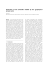sure which was identified as the ”atmospheric pole tide”. Plag (1997) showed the 14-16-month atmospheric pressure fluctuations are responsible for most of the oceanic pole tide attributed to the CW...

### EPF iprotect® (Ecological Pressure Filter)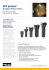### Aazevmonteiro Construções Exportações Importações Unipessoal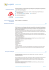### Programme Nov10_AAM programme Sept 2010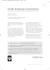### Nov/Dec 2012 Issue - Automobile Association of Malaysia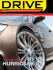### polar - Kinnarps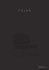### the renault clio rs - Automobile Association of Malaysia### Polar Space Task Group Overview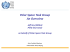### measuring - Amazon Web Services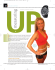### Optical Excitations in Biological Systems: Multiscale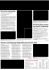### Germanium Drive Manual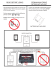### ABSTRACT Solubility and Spectroscopy of Unsaturated